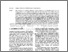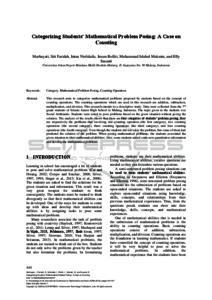# Categorizing students’ mathematical problem posing: A case on counting

Marhayati, Marhayati and Faridah, Siti and Nisfulaila, Intan and Rofiki, Imam and Mukmin, Muhammad Islahul and Susanti, Elly (2018) Categorizing students’ mathematical problem posing: A case on counting. Presented at 1st International Conference on Mathematics and Islam (ICMIs), 3-5 Aug 2018, Mataram, Indonesia.Preview
Text (Fulltext)
5325.pdf - Published Version

Full text available at: https://www.scitepress.org/PublicationsDetail.aspx...

## Abstract

This research aims to categorize mathematical problems proposed by students based on the concept of counting operations. The counting operations which are used in this research are addition, subtraction, multiplication, and division. This research intends to a descriptive study. Data were collected from the 7th grade students of Islamic Junior High School in Malang, Indonesia. The topic given to the students was Social Arithmetic. Students were asked to pose problems based on the given situation without giving the solution. The analysis of the results shows that there are four categories of students’ problem posing. They are respectively the problems that involving one counting operation (the first category), two counting operations (the second category), three counting operations (the third category), and four counting operations (the fourth category). Even though the students did not solve the problem, but some of them had predicted the solution of the problem. When posing mathematical problems, the students associated the given situation to their mathematical abilities. Also, some students added some new quantitative information and develop the mathematical problems.

Item Type: Conference (Paper) category; counting operation; mathematical problem posing 13 EDUCATION > 1301 Education Systems > 130106 Secondary Education Faculty of Tarbiyah and Teaching Training > Department of Teacher Education for Islamic Primary Schools Imam Rofiki 27 Mar 2020 00:35View Item